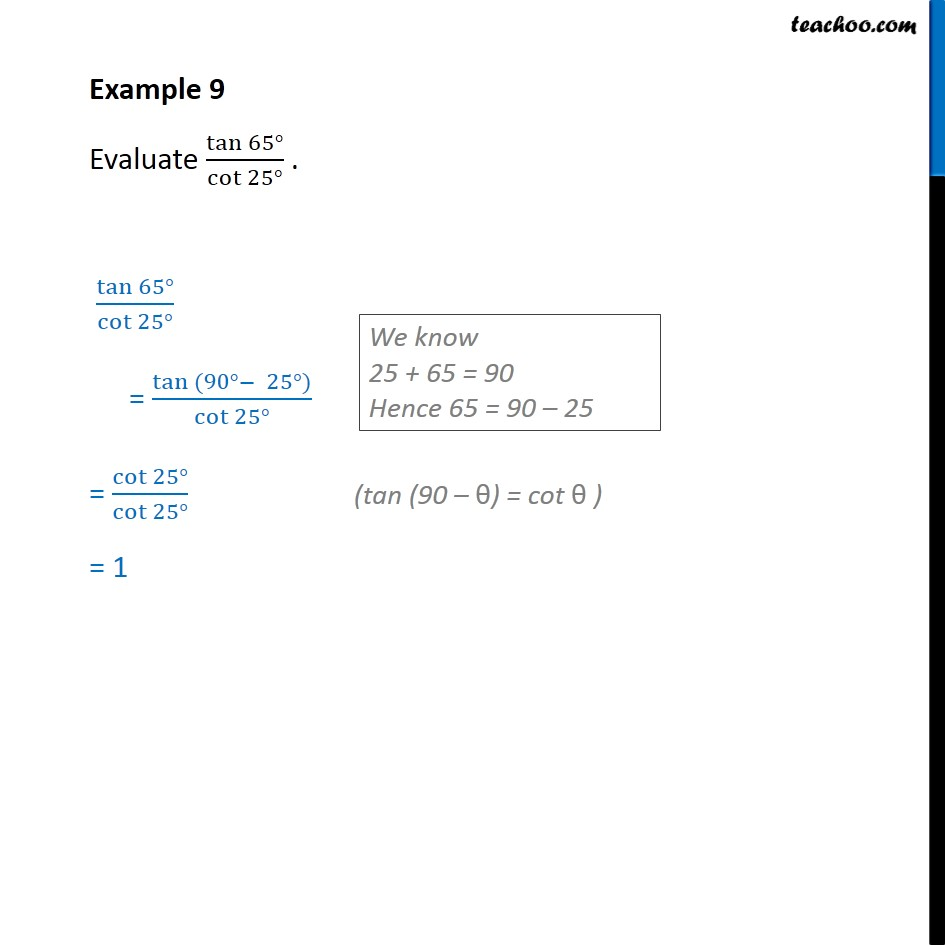1. Chapter 8 Class 10 Introduction to Trignometry (Term 1)
2. Serial order wise
3. Examples

Transcript

Example 9 Evaluate tan 65° /cot 25° . Now, tan 65 / cot 25 We know that 25 + 65 = 90 Hence, 65 = 90 - 25 So, we put tan 65 as tan (90 - 25) = ( tan (90 - 25))/cot 25 Now, we know that tan (90 - θ) = cot θ = cot 25 /cot 25 = 1